Meta-analysis combines the results of multiple studies that answer similar research questions. Does exercise prolong life? Does lack of sleep increase the risk of cancer? Does daylight saving save energy? And more. Many studies attempt to answer such questions, and some report inconclusive or even conflicting results. Meta-analysis helps aggregate the information, often overwhelming, from many studies in a principled way into one unified final conclusion or provides the reason why such a conclusion cannot be reached.

Stata has a long history of meta-analysis methods contributed by Stata researchers, for instance, Palmer and Sterne (2016). Stata now offers the new suite of commands, meta, to perform meta-analysis. The new suite is broad, yet one of its strengths is its simplicity.

Let’s quickly look at one possible workflow. Also see the summary of all features in Summary of features in four tables and more examples in Let’s see it work.

Quick workflow

Obtain meta-analysis summary

Explore heterogeneity

Investigate small-study effects and publication bias

Tell meta that effect sizes and their standard errors are already stored in variables such as es and se,

```. meta set es se
```

or that you have binary summary data and want to compute, for instance, log odds-ratios,

```. meta esize n11 n12 n21 n22, esize(lnoratio)
```

or that you have continuous summary data and want to compute, for instance, Hedges’s gstandardized mean differences,

```. meta esize n1 mean1 sd1 n2 mean2 sd2, esize(hedgesg)
```

Obtain meta-analysis summary. Estimate overall effect size and its CI, obtain heterogeneity statistics, and more:

```. meta summarize

```

Or produce a forest plot:

```. meta forestplot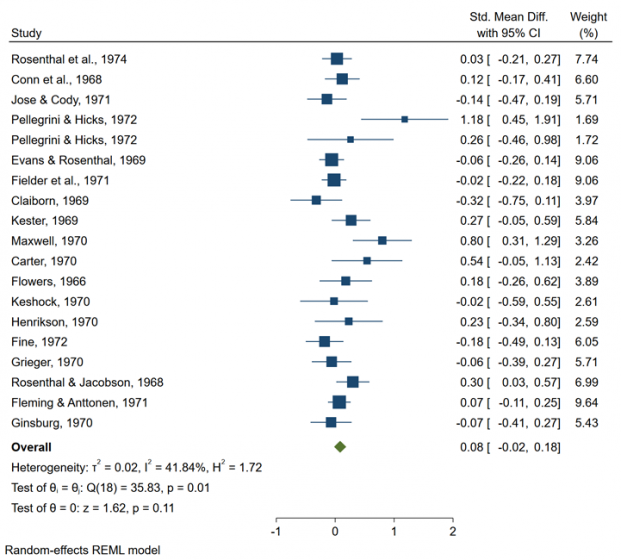```

Explore heterogeneity. Perform subgroup meta-analysis:

```. meta forestplot, subgroup(group)

```

Or meta-regression:

```. meta regress i.group x

```

Investigate small-study effects and publication bias. Produce a funnel plot:

```. meta funnelplot

```

Check whether funnel-plot asymmetry is due to publication bias using a contour-enhanced funnel plot:

```. meta funnelplot, contours(1 5 10)

```

Test formally for funnel-plot asymmetry:

```. meta bias, egger

```

Assess publication bias using the trim-and-fill method:

```. meta trimfill

```

Also see the summary of all features in Summary of features in four tables. For more examples, see Let’s see it work.

Summary of features in four tables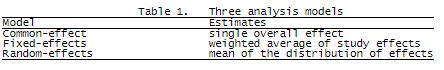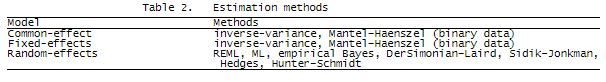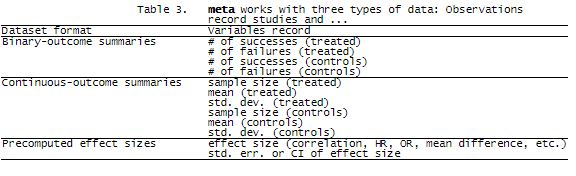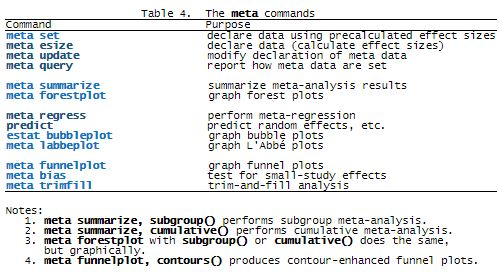Let’s see it work Example dataset:

Effects of teacher expectancy on pupil IQ

Meta-analysis summary

Forest plot

Heterogeneity

Summary measures and homogeneity test

Subgroup analysis

Meta-regression

Postestimation: bubble plots

Small-study effects and publication bias

Standard and contour-enhanced funnel plots

Tests for funnel-plot asymmetry

Trim-and-fill analysis

Cumulative meta-analysis

Example dataset: Effects of teacher expectancy on pupil IQ

To demonstrate the meta suite, we use the famous example from Raudenbush (1984) of the meta-analysis of 19 studies that evaluated the effects of teacher expectancy on pupil IQ. In their original study, Rosenthal and Jacobson (1968) discovered the so-called Pygmalion effect, in which expectations of teachers affected outcomes of their students.

The goal of the experiment was to investigate whether the identification of the randomly selected group of students (experimental group) to teachers as “likely to show dramatic intellectual growth” would influence teachers’ expectations for these students. The authors found a statistically significant effect between the experimental and control groups with respect to students’ IQ scores.

Later studies attempted to replicate the results, but many did not find the hypothesized effect. Raudenbush (1984) suspected that the Pygmalion effect might be mitigated by how long the teachers had worked with the students before the experiment.

We load the dataset and describe some of its variables below.

```. webuse pupiliq
(Effects of teacher expectancy on pupil IQ)

. describe studylbl stdmdiff se week1

```
 storage display value variable name type format label variable label studylbl str26 %26s Study label stdmdiff double %9.0g Standardized difference in means se double %10.0g Standard error of stdmdiff week1 byte %9.0g catweek1 Prior teacher-student contact > 1 week

stdmdiff and se record precomputed effect sizes, standardized mean differences between the experimental and control groups, and their standard errors.

studylbl contains study labels, which include the authors and publication years.

weeks records the number of weeks of prior contact between the teacher and the students. week1 is the dichotomized version of weeks, which records the high-contact (week1=1) and low-contact (week1=0) groups.

Declaring the meta-analysis data is the first step of your meta-analysis in Stata. During this step, you specify the main information needed for meta-analysis such as the study-specific effect sizes and their standard errors. You declare this information once by using either meta set or meta esize, and it is then used by all meta commands. The declaration step helps minimize potential mistakes and typing; see [META] meta data for details.

Let’s declare our pupil IQ data. Our dataset contains already calculated effect sizes (stdmdiff) and their standard errors (se), so we use meta set for declaration. If you have study-specific summary data and want to compute effect sizes, see meta esize.

```. meta set stdmdiff se, studylabel(studylbl) eslabel(Std. Mean Diff.)

```
 Meta-analysis setting information Study information No. of studies: 19 Study label: studylbl Study size: N/A Effect size Type: Generic Label: Std. Mean Diff. Variable: stdmdiff Precision Std. Err.: se CI: [_meta_cil, _meta_ciu] CI level: 95% Model and method Model: Random-effects Method: REML

We also specified how we want the meta commands to label studies and effect sizes in the output.

In addition to our specifications, meta set reported other settings that will be used by meta by default such as those for the meta-analysis model and method. meta‘s default is a random-effects model with the REML estimation method, but you can specify any other of the supported methods; see Declaring a meta-analysis model. Also see [META] meta data for more information about how to declare the meta-analysis data.

After the declaration, you can use meta query or meta update to describe or update your current meta settings at any point of your meta-analysis.

## Meta-analysis summary

After the declaration, you can use any of the meta commands to perform meta-analysis.

For instance, we can use meta summarize to obtain basic meta-analysis summary results and display them in a table:

```. meta summarize

Effect-size label:  Std. Mean Diff.
Effect size:  stdmdiff
Std. Err.:  se
Study label:  studylbl

Meta-analysis summary                              Number of studies =     19
Random-effects model                               Heterogeneity:
Method: REML                                                   tau2 =  0.0188
I2 (%) =   41.84
H2 =    1.72

Effect Size: Std. Mean Diff.```
 Study Effect Size [95% Conf. Interval] % Weight Rosenthal et al., 1974 0.030 -0.215 0.275 7.74 Conn et al., 1968 0.120 -0.168 0.408 6.60 Jose & Cody, 1971 -0.140 -0.467 0.187 5.71 Pellegrini & Hicks, 1972 1.180 0.449 1.911 1.69 Pellegrini & Hicks, 1972 0.260 -0.463 0.983 1.72 Evans & Rosenthal, 1969 -0.060 -0.262 0.142 9.06 Fielder et al., 1971 -0.020 -0.222 0.182 9.06 Claiborn, 1969 -0.320 -0.751 0.111 3.97 Kester, 1969 0.270 -0.051 0.591 5.84 Maxwell, 1970 0.800 0.308 1.292 3.26 Carter, 1970 0.540 -0.052 1.132 2.42 Flowers, 1966 0.180 -0.257 0.617 3.89 Keshock, 1970 -0.020 -0.586 0.546 2.61 Henrikson, 1970 0.230 -0.338 0.798 2.59 Fine, 1972 -0.180 -0.492 0.132 6.05 Grieger, 1970 -0.060 -0.387 0.267 5.71 Rosenthal & Jacobson, 1968 0.300 0.028 0.572 6.99 Fleming & Anttonen, 1971 0.070 -0.114 0.254 9.64 Ginsburg, 1970 -0.070 -0.411 0.271 5.43 theta 0.084 -0.018 0.185
```Test of theta = 0: z = 1.62                                Prob > |z| = 0.1052
Test of homogeneity: Q = chi2(18) = 35.83                    Prob > Q = 0.0074
```

Or we can use meta forestplot to produce results on a forest plot:

`. meta forestplot`
 Effect-size label: Std. Mean Diff. Effect size: stdmdiff Std. Err.: se Study label: studylblBoth commands include the information about study-specific effect sizes and their CIs, in addition to the estimate of the overall effect size and its CI. For instance, from the plot, the estimated overall standardized mean difference is 0.08 with a 95% CI of [-0.02, 0.18].

Various heterogeneity measures and tests are also reported; we explore them below in Heterogeneity.

For more interpretation of the results, see Basic meta-analysis summary in [META] meta. For details about the commands, see [META] meta summarizeand [META] meta forestplot.

Heterogeneity

In meta-analysis, heterogeneity occurs when variation between the study effect sizes cannot be explained by sampling variability alone. meta summarizeand meta forestplot report basic heterogeneity measures and the homogeneity test to assess the presence of heterogeneity.

When there are study-level covariates, also known as moderators, that may explain some of the between-study variability, heterogeneity can be explored further via subgroup analysis and, more generally, via meta-regression. Subgroup analysis is used with categorical moderators, and meta-regression is used when at least one of the moderators is continuous.

Summary measures and homogeneity test

Subgroup analysis

Meta-regression

Postestimation: Bubble plot

### Summary measures and homogeneity test

Consider the forest plot we produced in Meta-analysis summary.The between-study variation of the effect sizes is evident from the forest plot. The reported heterogeneity statistics indicate the presence of heterogeneity in these data. For instance,  is estimated to be 41.84%, which, according to Higgins et al. (2003), indicates the presence of “medium heterogeneity”.

The test of homogeneity of study-specific effect sizes is also rejected, with a chi-squared test statistic of 35.83 and a p-value of 0.01.

### Subgroup analysis

Subgroup analysis is used when study effect sizes are expected to be more homogeneous within certain groups. The grouping variables can be specified in option subgroup() supported by meta summarize and meta forestplot.

In Summary measures and homogeneity test, we established the presence of heterogeneity between the study results. As we said in Example dataset: Effects of teacher expectancy on pupil IQ, it was suspected that the amount of contact between the teachers and students before the experiment may explain some of the between-study variability.

Let’s first consider the binary variable week1 that divides the studies into the high-contact (week1=1) and low-contact (week1=0) groups. (Below Meta-regression, we explore the impact of continuous weeks on the effect sizes.)

For categorical variables, we can perform subgroup analysis—separate meta-analysis for each group—to explore heterogeneity between the groups.

`. meta forestplot, subgroup(week1)`
 Effect-size label: Std. Mean Diff. Effect size: stdmdiff Std. Err.: se Study label: studylblOption subgroup() can be used with meta forestplot and meta summarize to perform subgroup analysis. In our example, we specified only one grouping variable, week1, but you can include more, provided you have a sufficient number of studies per group.

After stratifying on the contact group, the results appear to be more homogeneous, particularly within the high-contact group (> 1 week). The test of no differences between the groups, reported at the bottom of the graph, is rejected with a chi-squared test statistic of 14.77 and a p-value less than 0.01.

Also see Subgroup meta-analysis in [META] meta.

### Meta-regression

Meta-regression is often used to explore heterogeneity induced by the relationship between moderators and study effect sizes. Moderators may include a mixture of continuous and categorical variables. In Stata, you perform meta-regression by using meta regress.

Continuing with our heterogeneity analysis, let’s use meta-regression to explore the relationship between study-specific effect sizes and the amount of prior teacher–student contact (weeks).

```. meta regress weeks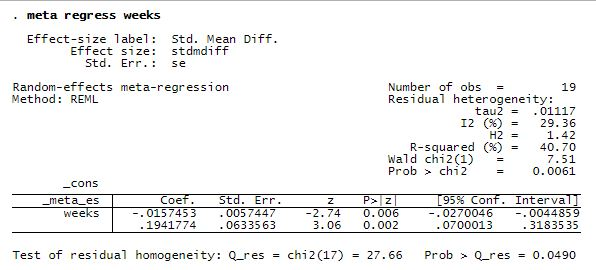```
```
There is a statistically significant negative relationship between the magnitudes of the effect sizes and the number of weeks of prior contact: the more time teachers spent with students before the experiment, the smaller the estimated effect size.Test of residual homogeneity: Q_res = chi2(17) = 27.66   Prob > Q_res = 0.0490
```

After accounting for weeks, we find that the remaining between-study residual heterogeneity is roughly 30%.

Also see Heterogeneity: Meta-regression and bubble plot in [META] meta and [META] meta regress.

Postestimation: Bubble plot

Continuing with Meta-regression, we can produce a bubble plot after meta-regression with one continuous covariate to explore the relationship between the effect sizes and the covariate.

```. estat bubbleplot
```The standardized mean difference decreases as the number of weeks of prior teacher–student contact increases. There are also several outlying studies in the region where weeks is less than roughly 3 weeks. The size of the bubbles represents the precision of the studies. Some of the outlying studies also appear to be among the more precise studies.

Also see Heterogeneity: Meta-regression and bubble plot in [META] meta[META] estat bubbleplot, and, more generally, [META] meta regress postestimation.

Small-study effects and publication bias

The term “small-study effects” refers to situations where the effects of smaller studies differ systematically from the effects of larger studies. For instance, smaller studies may report larger effect sizes than larger studies. Two common reasons for the presence of small-study effects are between-study heterogeneity and publication bias.

Publication bias arises when the decision of whether to publish a study’s results depends on the significance of the obtained results. Often, smaller studies with nonsignificant findings are suppressed from publication. This may lead to a biased sample of studies in a meta-analysis, which is often collected from the published studies.

The meta suite provides three commands you can use to explore small-study effects and publication bias.

meta funnelplot produces standard and contour-enhanced funnel plots, which can be used to explore small-study effects and publication bias visually.

meta bias provides several statistical tests for small-study effects, also known as tests for funnel-plot asymmetry.

meta trimfill performs nonparametric trim-and-fill analysis that explores the sensitivity of the meta-analysis results to potentially omitted studies.

We demonstrate these commands in what follows.

Standard and contour-enhanced funnel plots

Tests for funnel-plot asymmetry

Trim-and-fill analysis

### Standard and contour-enhanced funnel plots

To demonstrate, let’s produce a funnel plot for the pupil IQ data.

`. meta funnelplot`
 Effect-size label: Std. Mean Diff. Effect size: stdmdiff Std. Err.: se Model: Common-effect Method: Inverse-varianceIn the absence of publication bias and, more generally, small-study effects, the funnel plot should resemble a symmetric inverted funnel. In our example, it appears that a few points (studies) are missing in the lower left portion of the funnel plot, which makes it look asymmetric.

Recall, however, that in our earlier heterogeneity analysis, we established the presence of between-study variability. Thus, this may be one of the reasons for the asymmetry of the funnel plot.

Contour-enhanced funnel plots are often used to explore whether the funnel-plot asymmetry is due to publication bias or perhaps some other factors. Let’s add the 1%, 5%, and 10% significance contours to our funnel plot.

`. meta funnelplot, contours(1 5 10)`
 Effect-size label: Std. Mean Diff. Effect size: stdmdiff Std. Err.: se Model: Common-effect Method: Inverse-varianceBased on the contour-enhanced funnel plot, it appears that we are missing a few smaller studies that fall both in the significant and nonsignificant regions of the funnel plot. Under publication bias, we are likely to see missing smaller studies only in the nonsignificant regions. So, perhaps, the funnel-plot asymmetry in our example is due to some other reason such as heterogeneity.

In fact, the meta-analysis literature recommends that the heterogeneity be addressed before the exploration of the publication bias. For instance, in our example, we can produce funnel plots separately for each contact group.

`. meta funnelplot, by(week1)`
 Effect-size label: Std. Mean Diff. Effect size: stdmdiff Std. Err.: se Model: Common-effect Method: Inverse-varianceWithin each contact group, funnel plots look more symmetric.

Also see Funnel plots for exploring small-study effects in [META] meta and [META] meta funnelplot.

Tests for funnel-plot asymmetry

Continuing with Standard and contour-enhanced funnel plots, we can use one of the statistical tests to test formally for the funnel-plot asymmetry. These are also known as tests for small-study effects.

Let’s use the Egger regression-based test to test for the funnel-plot asymmetry in the pupil IQ data.

`. meta bias, egger`
 Effect-size label: Std. Mean Diff. Effect size: stdmdiff Std. Err.: se Regression-based Egger test for small-study effects Random-effects model Method: REML H0: beta1 = 0; no small-study effects beta1 = 1.83 SE of beta1 = 0.724 z = 2.53 Prob > |z| = 0.0115

The null hypothesis of no small-study effects or, equivalently, of the symmetry of the funnel plot is rejected at the 5% significance level with a z statistic of 2.53 and a p-value of 0.0115.

But, if we account for the between-study heterogeneity due to week1, the results of the test are no longer statistically significant.

`. meta bias week1, egger`
 Effect-size label: Std. Mean Diff. Effect size: stdmdiff Std. Err.: se Regression-based Egger test for small-study effects Random-effects model Method: REML Moderators: week1 H0: beta1 = 0; no small-study effects beta1 = 0.30 SE of beta1 = 0.729 z = 0.41 Prob > |z| = 0.6839

Also see Testing for small-study effects in [META] meta and [META] meta bias.

`Trim-and-fill analysis`

In the presence of publication bias, it is useful to explore its impact on the meta-analysis results. One way to do this is to perform trim-and-fill analysis.

In Standard and contour-enhanced funnel plots and Tests for funnel-plot asymmetry, we detected the asymmetry of the funnel plot but commented that this may be because of heterogeneity rather than publication bias. In fact, the contour-enhanced funnel plot suggested that the asymmetry is likely not because of publication bias. But, for the purpose of this demonstration, let’s go ahead and pretend that the observed asymmetry in the funnel plot is induced by publication bias and that we want to explore its impact on our meta-analysis results.

```. meta trimfill, funnel

Effect-size label:  Std. Mean Diff.
Effect size:  stdmdiff
Std. Err.:  se

Nonparametric trim-and-fill analysis of publication bias
Linear estimator, imputing on the left

Iteration                            Number of studies =     22
Model: Random-effects                       observed =     19
Method: REML                                  imputed =      3

Pooling
Model: Random-effects
Method: REML```
 Studies Std. Mean Diff. [95% Conf. Interval] Observed 0.084 -0.018 0.185 Observed + Imputed 0.028 -0.117 0.173meta trimfill estimated the number of studies missing presumably due to publication bias to be 3, imputed the omitted studies, and reported additional results using both the observed and imputed studies. With the imputed studies, the overall effect-size estimate is reduced from 0.084 to 0.028 with a wider 95% CI.

We also specified option funnel to produce the funnel plot that includes the omitted studies. The imputed studies make the funnel plot look more symmetric and identify the areas where studies are missing.

Given the presence of heterogeneity, however, we should have addressed it first before the trim-and-fill analysis. For instance, we could have run meta trimfill separately for low-contact and high-contact groups.

Also see Trim-and-fill analysis for addressing publication bias in [META] meta and [META] meta trimfill.

`Cumulative meta-analysis`

In Meta-regression, we established that there is a negative association between the magnitudes of effect sizes and the amount of prior teacher–student contact (weeks). We can perform cumulative meta-analysis to explore the trend in the effect sizes as a function of weeks. We display the results as a forest plot.

`. meta forestplot, cumulative(weeks)`
 Effect-size label: Std. Mean Diff. Effect size: stdmdiff Std. Err.: se Study label: studylblWe specified weeks in meta forestplot‘s option cumulative() to perform cumulative meta-analysis with weeks as the ordering variable. This option is also supported by meta summarize.

The studies are first ordered with respect to weeks, from smallest to largest amount of contact. Then, separate meta-analyses are performed by adding one study at a time. That is, the first result of the cumulative forest plot corresponds to the effect size and its CI from the first study. The second result corresponds to the overall effect size and its CI from the meta-analysis of the first two studies. And so on. The last result corresponds to the standard meta-analysis using all studies.

As the number of weeks increases, the overall standardized mean difference and its significance (p-value) decreases.